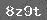# 第三册期中达标自测题

（时间：60分钟100分）

35843     3×310

551827   1936-27

5×530     93-5826

71324    4×415

31-243×2  80-6420

8÷42  5416-40=

3×12=   70-35+18=

42×3=    50-14+ 17=

10÷54=   80-1521=

6×24=    2130- 22=

1．看算式填空：

2 ×4=   表示□个□

4×2=   表示□个□

15÷3=  15里面有□个3

20÷4=  20里面有□个4

24÷6=  24里面有□个6

2．看图填空：1．在□中最大能填的数是几？

3×□＜5     □×415

□×635   5×□<20

4×□＜10    6×□＜20

□×15    □×211

  下一页

### 相关推荐

 留言与评论（共有 0 条评论）

 验证码：